International
Tables for
Crystallography
Volume B
Reciprocal space
Edited by U. Shmueli

International Tables for Crystallography (2010). Vol. B, ch. 1.3, pp. 89-91   | 1 | 2 |

## Section 1.3.4.3.6. Global crystallographic algorithms

G. Bricognea

aGlobal Phasing Ltd, Sheraton House, Suites 14–16, Castle Park, Cambridge CB3 0AX, England, and LURE, Bâtiment 209D, Université Paris-Sud, 91405 Orsay, France

#### 1.3.4.3.6. Global crystallographic algorithms

| top | pdf |

All the necessary ingredients are now available for calculating the CDFT for any given space group.

#### 1.3.4.3.6.1. Triclinic groups

| top | pdf |

Space group P1 is dealt with by the methods of Section 1.3.4.3.5.1andby those of Section 1.3.4.3.5.4.

#### 1.3.4.3.6.2. Monoclinic groups

| top | pdf |

A general monoclinic transformation is of the form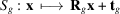witha diagonal matrix whose entries areor, anda vector whose entries are 0 or. We may thus decompose both real and reciprocal space into a direct sum of a subspacewhereacts as the identity, and a subspacewhereacts as minus the identity, with. All usual entities may be correspondingly written as direct sums, for instance: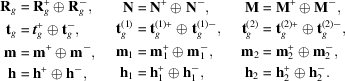We will use factoring by 2, with decimation in frequency when computing structure factors, and decimation in time when computing electron densities; this corresponds towith,. The nonprimitive translation vectorthen belongs to, and thusThe symmetry relations obeyed byand F are as follows: for electron densitiesor, after factoring by 2,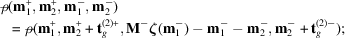while for structure factorswith its Friedel counterpartor, after factoring by 2,with Friedel counterpartWhen calculating electron densities, two methods may be used.

 (i) Transform onfirst. The partial vectors defined by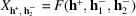obey symmetry relations of the formwithindependent of. This is the same relation as for the same parity class of data for a (complex or real) symmetricor antisymmetrictransform. The same techniques can be used to decrease the number ofby multiplexing pairs of such vectors and demultiplexing their transforms. Partial vectors with different values ofmay be mixed in this way (Section 1.3.4.3.5.6). Onceis completed, its results have Hermitian symmetry with respect to, and the methods of Section 1.3.4.3.5.1may be used to obtain the unique electron densities. (ii) Transform onfirst. The partial vectors defined by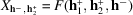obey symmetry relations of the formwithindependent of. This is the same relation as for the same parity class of data for a Hermitian symmetricor antisymmetrictransform. The same techniques may be used to decrease the number of. This generalizes the procedure described by Ten Eyck (1973)for treating dyad axes, i.e. for the case, and(simple dyad) or(screw dyad). Onceis completed, its results have Hermitian symmetry properties with respect towhich can be used to obtain the unique electron densities. Structure factors may be computed by applying the reverse procedures in the reverse order.

#### 1.3.4.3.6.3. Orthorhombic groups

| top | pdf |

Almost all orthorhombic space groups are generated by two monoclinic transformationsandof the type described in Section 1.3.4.3.6.2, with the addition of a centre of inversionfor centrosymmetric groups. The only exceptions are Fdd2 and Fddd which contain diamond glides, in which some nonprimitive translations are square roots' not of primitive lattice translations, but of centring translations. The generic case will be examined first.

To calculate electron densities, the unique octant of data may first be transformed on(respectively) as in Section 1.3.4.3.6.2using the symmetry pertaining to generator. These intermediate results may then be expanded by generatorby the formula of Section 1.3.4.3.3prior to the final transform on(respectively). To calculate structure factors, the reverse operations are applied in the reverse order.

The two exceptional groups Fdd2 and Fddd only require a small modification. The F-centring causes the systematic absence of parity classes with mixed parities, leaving only (000) and (111). For the former, the phase factorsin the symmetry relations of Section 1.3.4.3.6.2become powers of (−1) so that one is back to the generic case. For the latter, these phase factors are odd powers of i which it is a simple matter to incorporate into a modified multiplexing/demultiplexing procedure.

#### 1.3.4.3.6.4. Trigonal, tetragonal and hexagonal groups

| top | pdf |

All the symmetries in this class of groups can be handled by the generalized Rader/Winograd algorithms of Section 1.3.4.3.4.3, but no implementation of these is yet available.

In groups containing axes of the formwith g.c.d.along the c direction, the following procedure may be used (Ten Eyck, 1973):

 (i) to calculate electron densities, the unique structure factors indexed by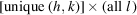are transformed on l; the results are rearranged by the transposition formula of Section 1.3.4.3.3so as to be indexed byand are finally transformed on (h, k) to produce an asymmetric unit. For a dihedral group, the extra twofold axis may be used in the transposition to produce a uniqueth of z. (ii) to calculate structure factors, the unique densities inth of z [orth for a dihedral group] are first transformed on x and y, then transposed by the formula of Section 1.3.4.3.3to reindex the intermediate results by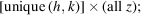the last transform on z is then carried out.

#### 1.3.4.3.6.5. Cubic groups

| top | pdf |

These are usually treated as their orthorhombic or tetragonal subgroups, as the body-diagonal threefold axis cannot be handled by ordinary methods of decomposition.

The three-dimensional factorization technique of Section 1.3.4.3.4.1allows a complete treatment of cubic symmetry. Factoring by 2 along all three dimensions gives four types (i.e. orbits) of parity classes: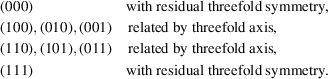Orbit exchange using the threefold axis thus allows one to reduce the number of partial transforms from 8 to 4 (one per orbit). Factoring by 3 leads to a reduction from 27 to 11 (in this case, further reduction to 9 can be gained by multiplexing the three diagonal classes with residual threefold symmetry into a single class; see Section 1.3.4.3.5.6). More generally, factoring by q leads to a reduction fromto. Each of the remaining transforms then has a symmetry induced from the orthorhombic or tetragonal subgroup, which can be treated as above.

No implementation of this procedure is yet available.

#### 1.3.4.3.6.6. Treatment of centred lattices

| top | pdf |

Lattice centring is an instance of the duality between periodization and decimation: the extra translational periodicity of ρ induces a decimation ofdescribed by the reflection conditions' on h. As was pointed out in Section 1.3.4.2.2.3, nonprimitive lattices are introduced in order to retain the same matrix representation for a given geometric symmetry operation in all the arithmetic classes in which it occurs. From the computational point of view, therefore, the main advantage in using centred lattices is that it maximizes decomposability (Section 1.3.4.2.2.4); reindexing to a primitive lattice would for instance often destroy the diagonal character of the matrix representing a dyad.

In the usual procedure involving three successive one-dimensional transforms, the loss of efficiency caused by the duplication of densities or the systematic vanishing of certain classes of structure factors may be avoided by using a multiplexing/demultiplexing technique (Ten Eyck, 1973):

 (i) for base-centred or body-centred lattices, two successive planes of structure factors may be overlaid into a single plane; after transformation, the results belonging to each plane may be separated by parity considerations; (ii) for face-centred lattices the same method applies, using four successive planes (the third and fourth with an origin translation); (iii) for rhombohedral lattices in hexagonal coordinates, three successive planes may be overlaid, and the results may be separated by linear combinations involving cube roots of unity.

The three-dimensional factorization technique of Section 1.3.4.3.4.1is particularly well suited to the treatment of centred lattices: if the decimation matrix of N contains as a factora matrix which integerizes' all the nonprimitive lattice vectors, then centring is reflected by the systematic vanishing of certain classes of vectors of decimated data or results, which can simply be omitted from the calculation. An alternative possibly is to reindex on a primitive lattice and use different representative matrices for the symmetry operations: the loss of decomposability is of little consequence in this three-dimensional scheme, although it substantially complicates the definition of the cocyclesand.

#### 1.3.4.3.6.7. Programming considerations

| top | pdf |

The preceding sections have been devoted to showing how the raw computational efficiency of a crystallographic Fourier transform algorithm can be maximized. This section will briefly discuss another characteristic (besides speed) which a crystallographic Fourier transform program may be required to possess if it is to be useful in various applications: a convenient and versatile mode of presentation of input data or output results.

The standard crystallographic FFT programs (Ten Eyck, 1973, 1985) are rather rigid in this respect, and use rather rudimentary data structures (lists of structure-factor values, and two-dimensional arrays containing successive sections of electron-density maps). It is frequently the case that considerable reformatting of these data or results must be carried out before they can be used in other computations; for instance, maps have to be converted from 2D sections to 3D bricks' before they can be inspected on a computer graphics display.

The explicitly three-dimensional approach to the factorization of the DFT and the use of symmetry offers the possibility of richer and more versatile data structures. For instance, the use of decimation in frequency' in real space and of decimation in time' in reciprocal space leads to data structures in which real-space coordinates are handled by blocks (thus preserving, at least locally, the three-dimensional topological connectivity of the maps) while reciprocal-space indices are handled by parity classes or their generalizations for factors other than 2 (thus making the treatment of centred lattices extremely easy). This global three-dimensional indexing also makes it possible to carry symmetry and multiplicity characteristics for each subvector of intermediate results for the purpose of automating the use of the orbit exchange mechanism.

Brünger (1989)has described the use of a similar three-dimensional factoring technique in the context of structure-factor calculations for the refinement of macromolecular structures.

### References

Brünger, A. T. (1989). A memory-efficient fast Fourier transformation algorithm for crystallographic refinement on supercomputers. Acta Cryst. A45, 42–50.
Ten Eyck, L. F. (1973). Crystallographic fast Fourier transforms. Acta Cryst. A29, 183–191.
Ten Eyck, L. F. (1985). Fast Fourier transform calculation of electron density maps. In Diffraction Methods for Biological Macromolecules (Methods in Enzymology, Vol. 115), edited by H. Wyckoff, C. H. W. Hirs & S. N. Timasheff, pp. 324–337. New York: Academic Press.